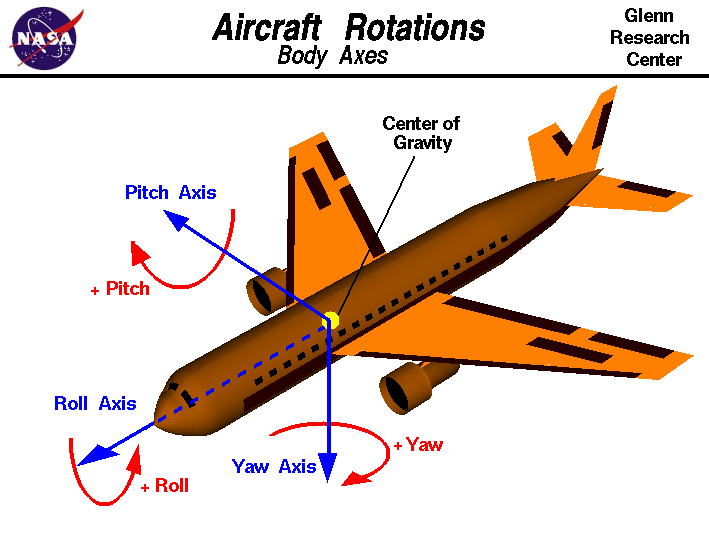# Simplified aerodynamics for 3D airplane

I'm looking how to implement simple movement for an airplane in 3D in godot, i couldn't find any tutorial or any hints on how to do this, mostly theoric information on a more real flight movement.

Any ideas on how to implement this?

I'm not looking for realistic physics, just a more arcade type of movement

• @a_donda I'm not looking for realistic physics, just a more arcade type of movement – Progs Nov 4 '20 at 15:13

Since you're looking for an arcade type of movement, I will make simplifications that will suit your use case. I will assume that you're familiar with the second law of Newton, as well as integration to compute speed and position based on acceleration.

An airplane in the air have 6 degrees of freedom: [X,Y,Z] are the coordinates in space (position) and the pitch, roll and yaw are the orientation around the plane's center of gravity.(Notice the axis orientation, in particular the Z axis pointing down, they are standard in aeronautics)

Planes in the air are subject to 4 forces: lift, weight, thrust and friction (airdrag). The resultant of these forces determines the moment and overall force that will accelerate and turn the airplane.Weight
The well-known formula $$\W = m.g\$$

Thrust
In your simplify model, it could be a constant multiplied by a user input.

Drag
In reality: $$\\frac{1}{2}.\rho.S.V^2.C_x\$$ With

• $$\\rho\$$ the air density
• $$\S\$$ the equivalent surface of your plane (more wing surface -> more drag and lift)
• $$\V\$$ the plane airspeed (speed relative to the air: a plane at 100 kts with a front wind of 20 kts has an airspeed of 120 kts)
• $$\C_x\$$ the drag coefficient depending on the plane geometry and the angle of incidence

In your simplified model: $$\A.V^2\$$ with A a well-tuned constant and V the airspeed of your plane.

Lift
In reality: $$\\frac{1}{2}.\rho.S.V^2.C_y\$$
In your simplified model : $$\A.V^2\$$ with A a well-tuned constant and V the airspeed of your plane. Note that drag and lift are very similar. The coefficients $$\C_x\$$ and $$\C_y\$$ are related and depend on the airplane geometry as well as the angle of incidence, but your simple model does not need that.

It's enough to compute easily the trajectory of the plane, but what about orientation? You've got to know the point of application of these forces relative to the center of gravity of your plane.
However, in arcade physics, the angular acceleration could be as simple as a constant multiplied by a user input. Integrated twice over time, that would give the angular position. Your job will be to tune the constants to achieve a desired behavior.

• what about pitch, yaw and roll? those don't require any calculation? – Progs Nov 18 '20 at 17:29
• Those are the 3 orientation variables, I've talked about them in the last paragraph. – Sacha Nov 18 '20 at 18:13